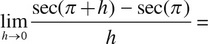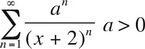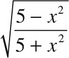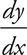# AP Calculus BC Practice Test 2

### Test Information9 questions18 minutes

Calculator Disallowed

1.2. Use differentials to approximate the change in the volume of a cube when the side is decreased from 8 to 7.99 cm (in cm3).

3. The radius of convergence ofis

4.(x) dx =

5. The equation of the line normal to y =at x = 2 is

6. If c satisfies the conclusion of the Mean Value Theorem for derivatives for f(x) = 2sin x on the interval [0, π], then c could be

7. The average value of f(x) = xln x on the interval [1, e] is

8. A 17-foot ladder is sliding down a wall at a rate of -5 feet/sec. When the top of the ladder is 8 feet from the ground, how fast is the foot of the ladder sliding away from the wall (in feet/sec)?

9. If= 3ycos x, and y = 8 when x = 0, then y =